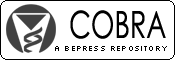## COBRA Preprint Series

#### Abstract

A general function to quantify the weight of evidence in a sample of data for one hypothesis over another is derived from the law of likelihood and from a statistical formalization of inference to the best explanation. For a fixed parameter of interest, the resulting weight of evidence that favors one composite hypothesis over another is the likelihood ratio using the parameter value consistent with each hypothesis that maximizes the likelihood function over the parameter of interest. Since the weight of evidence is generally only known up to a nuisance parameter, it is approximated by replacing the likelihood function with a reduced likelihood function on the interest parameter space. Unlike the Bayes factor and unlike the p-value under interpretations that extend its scope, the weight of evidence is coherent in the sense that it cannot support a hypothesis over any hypothesis that it entails. Further, when comparing the hypothesis that the parameter lies outside a non-trivial interval to the hypothesis that it lies within the interval, the proposed method of weighing evidence almost always asymptotically favors the correct hypothesis under mild regularity conditions. Even at small sample sizes, replacing a simple hypothesis with an interval hypothesis substantially reduces the probability of observing misleading evidence. Sensitivity of the weight of evidence to hypotheses' specification is mitigated by making them imprecise. The methodology is illustrated in the multiple comparisons setting of gene expression microarray data, and issues with simultaneous inference and multiplicity are addressed.

#### Disciplines

Bioinformatics | Computational Biology | Microarrays | Statistical Methodology | Statistical Theory

COinS